# Operations on Sets

Sets can be combined to form number of different sets.

The three basic operations on sets are:

1. Union of Sets

2. Intersection of Sets

3. Difference of Sets

# Union of Sets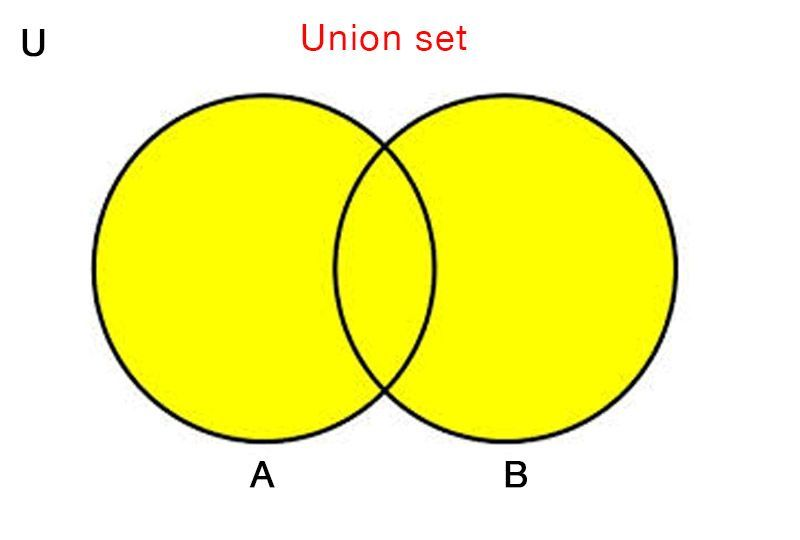The union of set A and set B is denoted by A∪B .

It contains those elements that are either in set A or in set B, or in both.

Union of sets is commutative. i.e. A∪B = B∪A.

Set A and set B are subsets of A∪B. i.e. A ⊂ (A∪B) and B ⊂ (A∪B)

# Intersection of Sets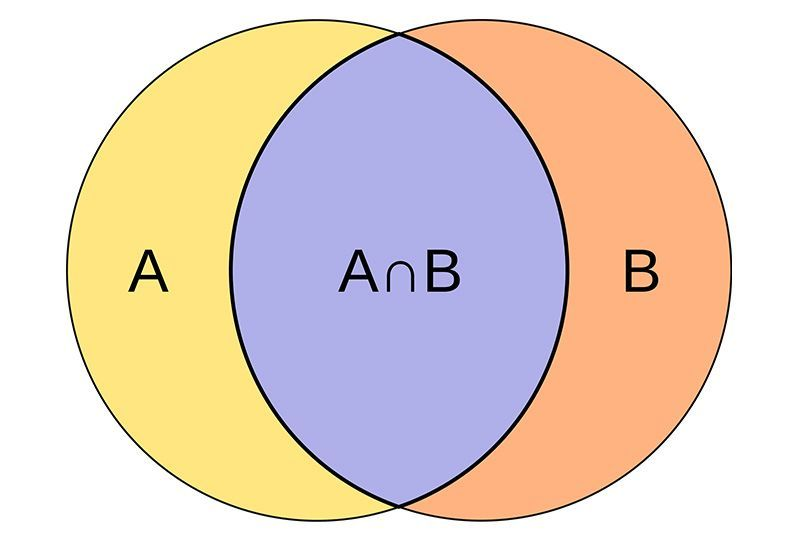The intersection of two sets is a set containing all the elements which are both in set A and in set B.

The intersection of two sets A and B is denoted by A∩B. Intersection of sets is commutative.

A∩B is a subset of set A and set B. i.e. (A∩B) ⊂ A and (A∩B) ⊂ B.

If A and B are disjoint sets, then A∩B = ∅ (null set)

# Difference of Sets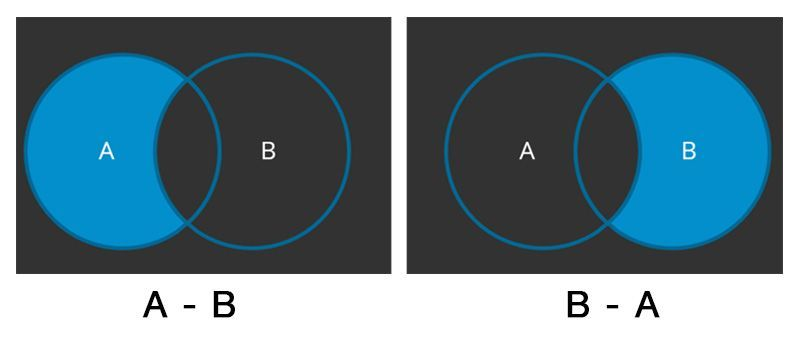The difference of two sets (A - B) is the set containing only those elements which are in set A and not in set B.

The difference of two sets A and B is represented as (A-B).

Difference of sets is not commutative. A - B ≠ B - A.

# Complement of Set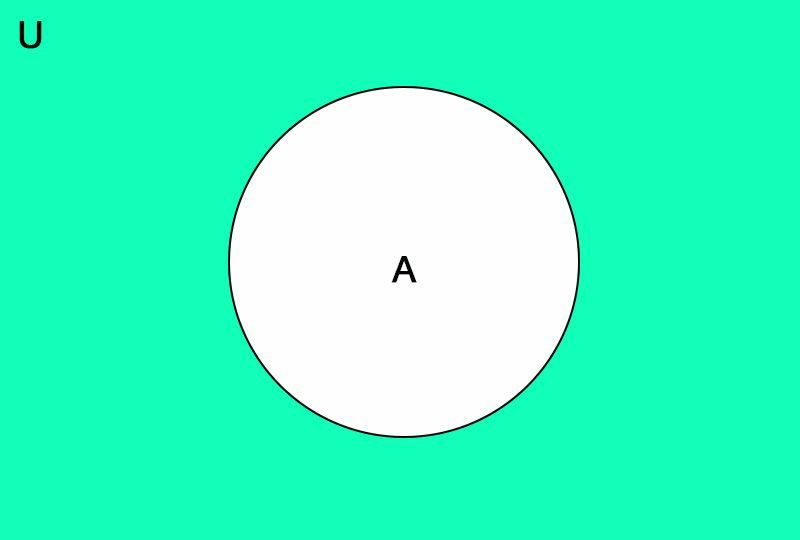The difference of the universal set and a set A is called the complement of the set A.

It is denoted as A'.

# Access All Content

## More from Grade 8 Math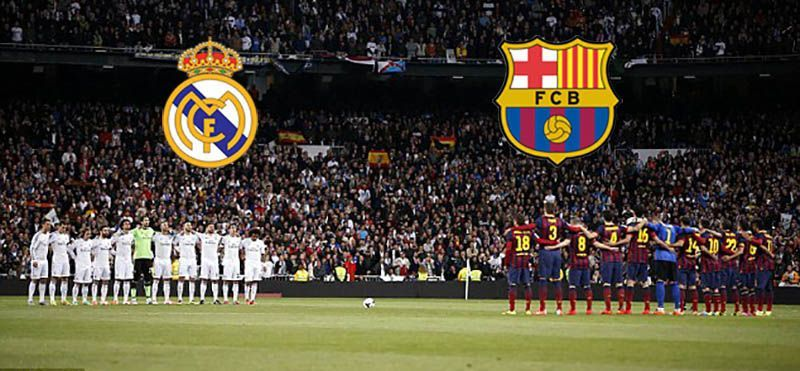# Sets

Sets are around us, everywhere. Learn how they are formed, named, and represented.# Celsius to Fahrenheit

Learn how to check the temperature, anytime and anywhere.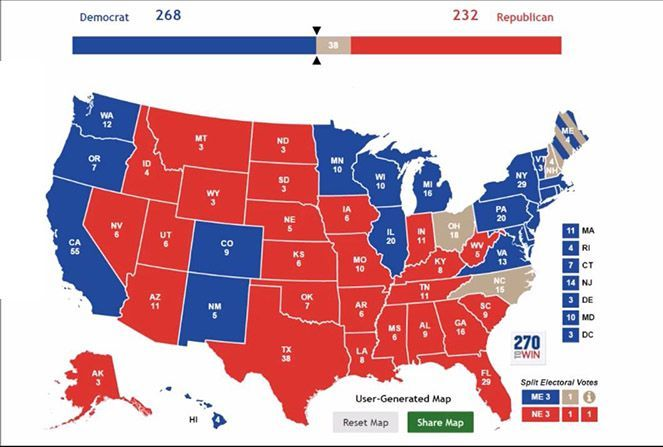# Sets and Operations

Learn about sets and its operations and see how Donald Trump won the election to become the US President.